# differential geometry

(redirected from Differential geometry and topology)
Also found in: Dictionary, Wikipedia.

## differential geometry,

branch of geometrygeometry
[Gr.,=earth measuring], branch of mathematics concerned with the properties of and relationships between points, lines, planes, and figures and with generalizations of these concepts.
in which the concepts of the calculuscalculus,
branch of mathematics that studies continuously changing quantities. The calculus is characterized by the use of infinite processes, involving passage to a limit—the notion of tending toward, or approaching, an ultimate value.
are applied to curves, surfaces, and other geometric entities. The approach in classical differential geometry involves the use of coordinate geometry (see analytic geometryanalytic geometry,
branch of geometry in which points are represented with respect to a coordinate system, such as Cartesian coordinates, and in which the approach to geometric problems is primarily algebraic.
; Cartesian coordinatesCartesian coordinates
[for René Descartes], system for representing the relative positions of points in a plane or in space. In a plane, the point P is specified by the pair of numbers (x,y
), but more recently the methods of differential geometry have been applied in other areas of geometry, e.g., in projective geometryprojective geometry,
branch of geometry concerned with those properties of geometric figures that remain invariant under projection. The basic elements are points, lines, and planes, and the following statements are usually taken as assumptions: (1) two points lie in a unique
.

### The Analysis of Curves

If a point r moves along a curve at arc length s from some fixed point, then t = dr/ds is a unit tangent vector to the curve at r. The normal vector n is perpendicular to the curve at the point and indicates the direction of the rate of change of t, i.e., the tendency of r to bend in the plane containing both r and t, and the binormal vector b is perpendicular to both t and n and indicates the tendency of the curve to twist out of the plane of t and n.

These three vectors are related by the three formulas of the French mathematician Jean Frédéric Frenet, which are fundamental to the study of space curves: dt/ds = &kgr;n; dn/ds = −&kgr;t + τb; db/ds = −τn, where the constants &kgr; and τ are the curvature and the torsion of the curve, respectively. Of special interest are the curves called evolutes and involutes; the evolute of a curve is another curve whose tangents are the normals to the original curve, and an involute of a curve is a curve whose evolute is the given curve.

### The Analysis of Surfaces

In the analysis of surfaces, points on a surface may be described not only with respect to the three-dimensional coordinates of the space in which the surface is considered but also with respect to an intrinsic coordinate system defined in terms of a system of curves on the surface itself. The curves on the surface that locally represent the shortest distances between points on the surface are called geodesics; geodesics on a plane are straight lines. Tangent and normal vectors are also defined for a surface, but the relationships between them are more complex than for a space curve (e.g., a surface has a whole circle of unit vectors tangent to it at a given point).

The results of the theory of surfaces are expressed most easily in the notation of tensorstensor,
in mathematics, quantity that depends linearly on several vector variables and that varies covariantly with respect to some variables and contravariantly with respect to others when the coordinate axes are rotated (see Cartesian coordinates).
. It is found that the total, or Gaussian, curvature of a surface is a bending invariant, i.e., an intrinsic property of the surface itself, independent of the space in which the surface may be considered. Of particular importance are surfaces of constant curvature; planes, cylinders, cones, and other so-called developable surfaces have zero curvature, while the elliptic and hyperbolic planes of non-Euclidean geometrynon-Euclidean geometry,
branch of geometry in which the fifth postulate of Euclidean geometry, which allows one and only one line parallel to a given line through a given external point, is replaced by one of two alternative postulates.
are surfaces of constant positive and negative curvature, respectively.

### Development of Differential Geometry

Differential geometry was founded by Gaspard Monge and C. F. Gauss in the beginning of the 19th cent. Important contributions were made by many mathematicians during the 19th cent., including B. Riemann, E. B. Christoffel, and C. G. Ricci. This work was collected and systematized at the end of the century by J. G. Darboux and Luigi Bianchi. The importance of differential geometry may be seen from the fact that Einstein's general theory of relativityrelativity,
physical theory, introduced by Albert Einstein, that discards the concept of absolute motion and instead treats only relative motion between two systems or frames of reference.
is formulated entirely in terms of the differential geometry, in tensor notation, of a four-dimensional manifold combining space and time.

The following article is from The Great Soviet Encyclopedia (1979). It might be outdated or ideologically biased.

## Differential Geometry

a branch of geometry, in which geometric configurations are studied using the methods of mathematical analysis. The principal objects of differential geometry are arbitrary sufficiently smooth curves and surfaces of Euclidian space as well as families of curves and surfaces. Differential geometry is primarily concerned with local properties of geometric configurations, that is, properties which hold for arbitrarily small portions of a geometric configuration. However, differential geometry is also concerned with properties of geometric configurations in the large (for example, properties of closed, convex surfaces).

The geometric objects studied in differential geometry are generally subject to certain smoothness requirements. Specifically, one usually requires that the functions defining the given objects are at least twice continuously differentiable.

The essence of the methods of differential geometry that are used to determine the local properties of geometric configurations are best explained using as an example the local study of the shape of curves.

At each point M of a sufficiently smooth curve L we can construct a tangent line MT and osculating plane π (Figure 1). Tangent MT is the limiting position of the secant MN as the point N approaches M along the curve L. The osculating plane is the limiting position of the variable plane passing through the tangent MT and the point N as N approaches M along L. The tangent MT can also be thought of as the line closest to L near M. Similarly, the osculating plane is the plane closest to L near M.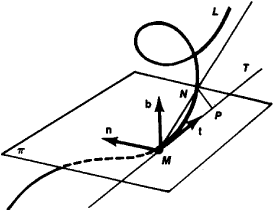Figure 1

To characterize geometrically the curvature of curve L near a given point M, we make use of the osculating circle, that is, the circle passing through M and closest to L near M. This means that if we consider values of only the first and second orders of smallness relative to the length of the arc MN, then a section of the curve L near M can be thought of as an arc of the osculating circle. The center of the osculating circle is called the center of curvature of L at the point M and its radius, the radius of curvature of L at M.

A numerical measure of the curvature k of L at M is the reciprocal 1/R of the radius R of the osculating circle: k = 1/R. The curvature k can also be viewed as a measure of the deviation of L from the tangent MT (Figure 1):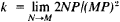or as the rate of change (rotation) of the tangent to L (Figure 2):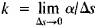where α is the angle between the tangents at the points M and N and Δ is the length of the arc MN.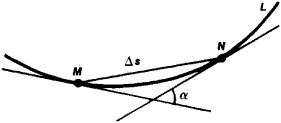Figure 2

Torsion σ serves as the measure of deviation of the curve from the osculating plane π at the point M; σ is defined as the limit of the ratio of the angle β between the osculating planes at the points M and N to the length Δs of the arc MN as Δs→0: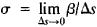The angle β is taken to be positive if an observer at M sees that the rotation of the osculating plane at N as N approaches M is counterclockwise. In the opposite case, β is taken to be negative. The torsion of the curve can be considered the rate of change (rotation) of the osculating plane. In particular, for a plane curve, the osculating plane coincides at all points with the surface of the curve, and, therefore, the torsion of such a curve at all points is equal to zero. The curvature k and the torsion Σ of a sufficiently smooth curve L are defined at each of its points and are functions of the parameter defining the points of this curve. To calculate k and Σ we must be given the curve in terms of suitable functions. Most frequently, the curve L is defined by parametric equations in rectangular coordinates:

(1) x = Ø(t), y = ψ(t), z = x(t)

As the parameter tchanges, the point M with coordinates (x, y, z) describes the curve L. In other words, parametric equations of a curve reflect the view of a curve as the trajectory of a moving point. The right-hand sides of equations (1) can be considered as projections on the coordinate axes of the radius vector r of the variable point M of the curve L. The vector r’ with coordinates {Ø)’(t), ψ’(t), x’(t)} is called the derivative of vector function r(t) and is directed along the tangent to L at point M.

Curvature and torsion are calculated according to the formulas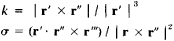where r’ x r” is the cross product and r’ • r” x r’” is the triple scalar product.

With each point M of curve L we associate three unit vectors—the tangent t, the principal normal n, and the binormal b (Figure 1). The vector n lies in the osculating plane and is directed from point M to the center of curvature of L at M. The vector b is orthogonal to t and n and is directed so that the vectors t, n, and b form a right-handed triple. This triple of vectors forms the so-called moving trihedral of the curve L. The surfaces of the vectors (n, b) and (t, b) are called the normal and rectifying planes of L at M, respectively.

The formulas for the derivatives of the vectors t, n, and b with respect to the length s of the arc L are called the Frenet formulas. They play a fundamental role in both the theory of curves and its applications (mechanics, theoretical physics, and so forth). The Frenet formulas are

dt/ds = kn

dn/ds = -kt + σh

db/ds = -σn

If the curvature and torsion are not equal to zero at a point M, then certain conclusions can be drawn about the shape of L near M: the projections of L onto the osculating and normal planes at M appear as shown in Figures 3 and 4, respectively. The shape of the projection onto the rectifying plane depends on the sign of the torsion. Projections of L onto a rectifying plane for σ > 0 and σ < 0 are shown in Figures 5 and 6. Curvature and torsion completely determine a curve. Specifically, if there exists a correspondence between the points of two curves such that the corresponding arcs of these curves are of equal length and, at the corresponding points, the curves have equal curvature and torsion, then these curves can be superposed on each other by means of a rigid motion.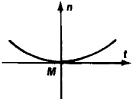Figure 3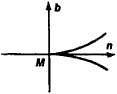Figure 4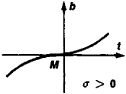Figure 5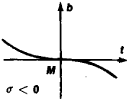Figure 6

The local study of curves suggests the manner of study of the local structure of a surface. At each point M of a sufficiently smooth surface S, we construct a tangent plane y and a uniquely defined osculating paraboloid π (Figure 7), which may degenerate into a parabolic cylinder or plane. The tangent plane may be viewed as the plane closest to 5 near M. The osculating paraboloid at M is characterized by the fact that, in a neighborhood of M, it coincides with S to within terms of the third order of smallness relative to the size of this neighborhood. Using osculating paraboloids, we classify the points M of a surface as elliptic (the osculating paraboloid is elliptic; Figure 8), hyperbolic (the osculating paraboloid is hyperbolic; Figure 9), parabolic (the osculating paraboloid is a parabolic cylinder; Figure 10), and planar (the osculating paraboloid is a plane; Figure 11).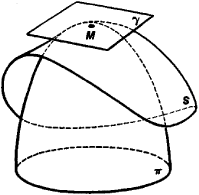Figure 7

In general, in studying the structure of a surface we make use of the so-called first and second fundamental quadratic forms of the surface. Thus, let S be a surface defined by the parametric equations

(2) x = Ø(u,v), y = ψ(u,v), z = x(u,v)

For a fixed value of v, equations (2) determine a curve on S, called a u-curve. A v-curve is determined in an analogous manner. The u- and v-curves are called parameter curves on S (if, for example, a sphere with a radius of 1 is defined by the parametric equations

x = cos u cos v, y = cos u sin v, z = sin u

then, the corresponding parameter curves are the meridians and parallels of this sphere). The quantities u and v are also called intrinsic coordinates, since a point on the surface is the point of intersection of the parameter curves passing through it, that is, it is determined by the parameter curves without reference to the space in which the sphere is embedded.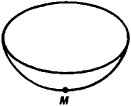Figure 8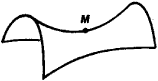Figure 9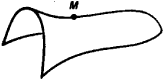Figure 10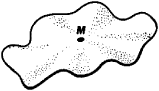Figure 11

The radius vector r of an arbitrary point M on S is determined by equations (2) as a function of u and v. The partial derivatives ru and rv of this function are vectors that are tangent to the u- and v-curves, respectively. These vectors at a point M lie in the tangent plane to S at M. The vector product [ru x rv] defines the normal to S at M.

Let s be the length of an arc of a curve L on S, and let u = f(t) and v = g(t) be its parametric equations in the intrinsic coordinates. Then, along L, r and s will be functions of t. The differential of s is determined by the equation ds2= dx2 + dy2 + dz2, the right-hand side of which is the square of the vector dr = vltdu + rrdv, that is, ds2= dr’2. Therefore,

ds2 = ru2du2 + 2rurududv + rv2dv2

Putting ru2 = E, ru rr = F, and rv2 = G, we can write ds2 as

The quadratic form on the right-hand side of (3) is called the first fundamental form of the surface S. Using this form, we can measure the lengths of arcs on 5 by integrating the expression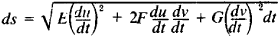along a given arc. Therefore, form (3) is also called the metric form of the surface. The first form also determines the intrinsic geometry of the surface, that is, the facts that can be determined by measurements carried out on the surface without reference to the space in which the surface is embedded. The intrinsic geometry of the surface does not change under bending, that is under a deformation of the surface thought of as an absolutely flexible and inextensible membrane.

The second fundamental form of a surface is given by the expression

Ldu2 + 2Mdudv + Ndv2

where L = ruun, M - ruv,n, and N = rvu (n is the unit vector of the normal to S at M. Using the second fundamental form, we can achieve an understanding of the spatial form of a surface. For example, the curvatures 1/R of normal sections of the surface at a given point M (that is, the curvatures of the curves of intersection of S with planes passing through the normal at M) are given by the expression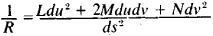The two fundamental forms of the surface, defined in terms of intrinsic coordinates, determine it up to position in space. Given two forms

Edu2 + 2Fdudv + Gdv2

and

Ldu2 + 2Mdudv + Ndv2

such that the first is positive definite and the coefficients L, M, and N of the second satisfy a certain system of equations of which one (obtained by K. Gauss) is algebraic and two others (obtained by K. M. Peterson) are linear partial differential equations of the first order, it is possible to find a surface for which these forms are the first and second fundamental forms, respectively.

The equations of Gauss and Peterson noted above play a fundamental role in theory of surfaces.

One of the objects of study in differential geometry are families of curves and surfaces. Such families are defined by equations containing parameters. For example, the equation (x - α)2+ y2= 1, containing the parameter α, defines a family of circles with a radius of 1 and with centers at (α, 0), that is, on the Ox axis (Figure 12).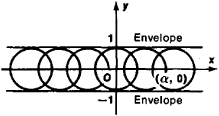Figure 12

By an envelope of a family of curves (surfaces) we mean a curve (surface) that is tangent to all curves (surfaces) of the family. In the above example the envelope consists of a pair of straight lines parallel to the Ox axis and at a distance of 1 from that axis. Two-parameter families of lines—congruences—have been studied in particular detail. The simplest example of a congruence is a family of parallel lines in space. Geometric optics is the source of the theory of congruences.

Various branches of differential geometry are devoted to the comprehensive study of differentiable manifolds. Examples of such manifolds are curves (one-dimensional manifolds), surfaces (two-dimensional manifolds), and ordinary Euclidian space (three-dimensional manifolds). A more complex example is the four-dimensional manifold, whose elements are lines of ordinary Euclidian space (a straight line in Cartesian coordinates is determined by equations of the type z = ax + b and z = cy + d; the numbers a, b, c, and d can be viewed as coordinates of this straight line).

The study of differentiable manifolds is being conducted in the following principal directions. (1) The geometry of a transitive group of mappings of a manifold onto itself, or the geometry of a “local group” of mappings. The subject matter includes classical local differential geometry (study of invariants of a group of motions of Euclidian space) as well as affine, projective, and conformal geometry (study of invariants of the corresponding group of transformations). (2) The geometry of manifolds with Riemannian metric (Riemannian spaces), which is a generalization to higher dimensions of the intrinsic geometry of surfaces viewed as two-dimensional Riemannian spaces. The geometry of Riemannian spaces plays an important role in the theory of relativity. (3) The geometry of so-called Finsler spaces, a generalization of Riemannian spaces. (4) The geometry of manifolds with connection, that is, manifolds in which we can compare geometric configurations in tangent spaces at different points.

The development of differential geometry is linked with the names of L. Euler and G. Monge. By the end of the 18th century they obtained important facts about the theory of surfaces. K. Gauss, who introduced both fundamental quadratic forms, made a significant contribution to the development of differential geometry. It was Gauss who proved the theorem about the invariance of the total curvature under isometric transformations. He actually laid the foundations for the intrinsic geometry of surfaces. The development of the foundations of the classical theory of surfaces was completed in the mid-19th century by K. M. Peterson, founder of the Moscow school of geometry. In the mid- and late 19th century, extensive and profound results in the classical theory of surfaces were obtained by F. Minding, J. Liouville, E. Beltrami, J. G. Darboux, and L. Bianchi. A number of remarkable results in classical differential geometry were obtained by the Russian scientists D. F. Egorov, N. N. Luzin, and S. P. Finikov.

The development of other directions in differential geometry is linked with the names of B. Riemann, G. Lamé, F. Klein, H. Weyl, and E. Cartan.

Various directions in differential geometry have been developed in the USSR. The greatest successes have been achieved in the study of problems in the large. (A. D. Aleksandrov, A. V. Pogorelov).

### REFERENCES

Monge, G. Prilozhenie analiza k geometrii. Moscow-Leningrad, 1936. (Translated from French.)
Struik, D. J. Ocherk istorii differentsial’noi geometrii do XX stoletiia. Moscow-Leningrad, 1941. (Translated from English.)
Pogorelov, A. V. Differentsial’naia geometriia, 5th ed. Moscow, 1969.
Rashevskii, P. K. Kurs differentsial’noi geometrii, 3rd ed. Moscow, 1950.
Blaschke, W. Vvedenie v differentsial’nuiu geometriiu. Moscow, 1957. (Translated from German.)
Rashevskii, P. K. Rimanova geometriia i tenzornyi analiz, 2nd ed. Moscow, 1964.
Aleksandrov, A. D. Vnutrenniaia geometriia vypuklykh poverkhnostei. Moscow-Leningrad, 1948.
Pogorelov, A. V. Vneshniaia geometriia vypuklykh poverkhnostei. Moscow, 1969.

E. G. POZNIAK